# Existence of partial derivatives not implies differentiable

## Statement

### For a function of two variables at a point

It is possible to have the following: a function$f$ of two variables$x,y$ and a point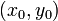$(x_0,y_0)$ in the domain of the function such that both the partial derivatives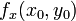$f_x(x_0,y_0)$ and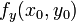$f_y(x_0,y_0)$ exist, but the gradient vector of$f$ at$(x_0,y_0)$ does not exist, i.e.,$f$ is not differentiable at$(x_0,y_0)$.

### For a function of two variables overall

It is possible to have the following: a function$f$ of two variables$x,y$ such that the partial derivatives$f_x(x,y)$ and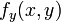$f_y(x,y)$ exist everywhere on$\R^2$, but the gradient vector$\nabla f$ is not defined everywhere, i.e., there is some point in$\R^2$ where$\nabla f$ does not exist.

### For a function of multiple variables

We can replace functions of two variables by functions of more than two variables in both the above formulations.

## Proof

### Example of a function for which the partial derivatives exist but it is not continuous

We give a single example that illustrates both versions of the statement.

Consider the function: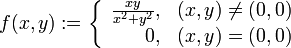$f(x,y) := \left\lbrace \begin{array}{rl} \frac{xy}{x^2 + y^2}, & (x,y) \ne (0,0) \\ 0, & (x,y) = (0,0) \\\end{array}\right.$

It's clear that$f$ is differentiable at all points other than$(0,0)$. At the point$(0,0)$, we note that:

• On the$x$-axis, the function is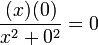$\frac{(x)(0)}{x^2 + 0^2} = 0$, i.e., it is identically the zero function along the$x$-axis. Thus,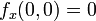$f_x(0,0) = 0$, i.e., the partial derivative exists and equals zero.
• On the$x$-axis, the function is$\frac{(0)(y)}{0^2 + y^2} = 0$, i.e., it is identically the zero function along the$y$-axis. Thus,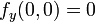$f_y(0,0) = 0$, i.e., the partial derivative exists and equals zero.

On the other hand,$f$ is not continuous at$(0,0)$. To see this, consider the limit along the line$y = x$. Setting$y = x$, we get that the limit is:$\lim_{x \to 0} \frac{xx}{x^2 + x^2} = \lim_{x \to 0} \frac{x^2}{2x^2} = \lim_{x \to 0}\frac{1}{2} = \frac{1}{2} \ne f(0,0)$

Note that if the function were indeed continuous at$(0,0)$, the limit along every direction would equal the value at the point, so this shows that the function is not continuous at$(0,0)$.

Since$f$ is not continuous at$(0,0)$, it cannot be differentiable at$(0,0)$. Another way of seeing the above computation is that since$f$ is not continuous along the direction$y = x$, the directional derivative along that direction does not exist, and hence$f$ cannot have a gradient vector.

### Idea behind example

The secret behind the example can be better understood using polar coordinates, though this is not necessary to understand the example itself. In polar coordinates, we can rewrite$f(x,y) = g(r,\theta)$ where:$g(r,\theta) = \left \lbrace \begin{array}{rl} \frac{1}{2}\sin(2\theta), & r \ne 0\\ 0, & r = 0 \\\end{array}\right.$

Here's how we get this. Plug in$x = r \cos \theta$ and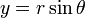$y = r \sin \theta$ in the original expression for points other than the origin. We have: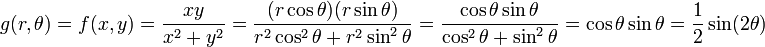$g(r,\theta) = f(x,y) = \frac{xy}{x^2 + y^2} = \frac{(r\cos \theta)(r \sin \theta)}{r^2 \cos^2\theta + r^2 \sin^2\theta} = \frac{\cos \theta \sin \theta}{\cos^2\theta + \sin^2\theta} = \cos \theta \sin \theta = \frac{1}{2}\sin(2\theta)$

• If$\theta$ is a multiple of$\pi/2$ (this includes the positive$x$-direction, positive$y$-direction, negative$x$-direction, and negative$y$-direction), the function is identically zero along the half-line corresponding to$\theta$.
• If$\theta$ is not a multiple of$\pi/2$, the function along the half-line corresponding to$\theta$ is the constant function with value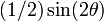$(1/2)\sin(2\theta)$, a nonzero number. In particular, it is discontinuous at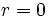$r = 0$, where the value suddenly jumps to zero. In particular, it is not differentiable along this direction.

### Example of a function where the partial derivatives exist and the function is continuous but it is not differentiable

Consider the multiplicatively separable function: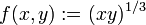$f(x,y) := (xy)^{1/3}$

We are interested in the behavior of$f$ at$(0,0)$.

This is slightly different from the other example in two ways. First, the partials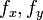$f_x, f_y$ do not exist everywhere, making it a worse example than the previous one. Second, the function is continuous at the point$(0,0)$ where both partial derivatives exist, and is still not differentiable, making it a better example than the previous one.

We first note that:

• For$x \ne 0$ and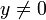$y \ne 0$, both partial derivatives$f_x, f_y$ exist. We have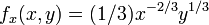$f_x(x,y) = (1/3)x^{-2/3}y^{1/3}$ and$f_y(x,y) = (1/3)x^{1/3}y^{-2/3}$.
• For points on the$x$-axis other than the origin (i.e., we have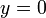$y = 0$),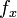$f_x$ exists and equals zero. This is because the function$f$ is identically zero along the$x$-axis. However,$f_y$ does not exist.
• For points on the$y$-axis other than the origin (i.e., we have$x = 0$),$f_y$ exists and equals zero. This is because the function$f$ is identically zero along the$y$-axis. However,$f_x$ does not exist.
• At the origin, both the partial derivatives$f_x,f_y$ exist and equal zero. This is because the function$f$ is identically zero along both the$x$- and the$y$-axis.

The upshot is that$f$ has partial derivatives at$(0,0)$.

However,$f$ is not differentiable at$(0,0)$. To see this, consider computing the directional derivative of$f$ along the line$y = x$, i.e., along the unit vector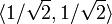$\langle 1/\sqrt{2},1/\sqrt{2} \rangle$. We get that the directional derivative is:$\lim_{h \to 0} \frac{((h/\sqrt{2})(h/\sqrt{2}))^{1/3} - 0}{h}$

The limit simplifies to: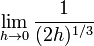$\lim_{h \to 0} \frac{1}{(2h)^{1/3}}$

The denominator approaches zero, so the limit expression is not finite. In particular, the directional derivative in this direction does not exist. The conclusion is that the function is not differentiable.